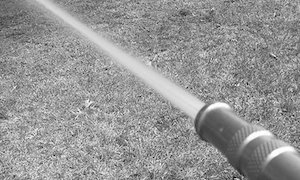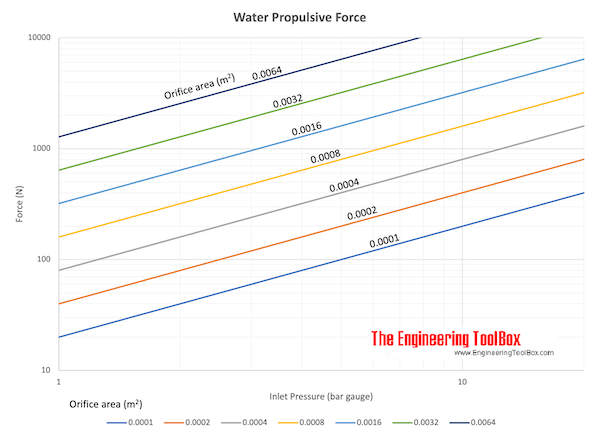Engineering ToolBox - Resources, Tools and Basic Information for Engineering and Design of Technical Applications!

# Jet Propulsion

## Calculate the propulsive discharge force or thrust induced by an incompressible jet flow.

The velocity out of a free jet can be expressed as

v2 = (2 (p1 - p2) / ρ)1/2                              (1)

where

v2 = velocity out of the jet (m/s)

p1 = pressure before the jet (N/m2, Pa)

p2 = ambient pressure after the jet (N/m2, Pa)

ρ = density of the fluid (kg/m3)

Note! - the density ρ is constant in incompressible flows and the equations are valid for liquids (like water) but not for gases (like air).

The flow volume out of a jet can be expressed as

q = A v2                                (2)

where

q = volume flow (m3/s)

A = orifice area of the jet (m2)

The propulsive force or thrust induced by the jet can be expressed as

F = ρ q (v2 - v1)                                (3)

where

v1 = jet velocity (m/s)

If the jet is not moving

v1 = 0

and (3) can be expressed as

F = ρ q v2                                    (3b)

- or alternatively with (2)

F = ρ A v22                                  (3c)

Substituting v2 with eq. (1) in eq. (3c) - the propulsive force or thrust induced by a jet not moving (v1 = 0) be expressed as

F = 2 A (p1 - p2)                                 (4)

### Example - Propulsive ForceWater flows through a garden hose with diameter 15 mm. The water pressure just before the outlet is 4 105 Pa (abs) and the atmospheric pressure is 1 105 Pa (abs).

The outlet area of the hose can be calculated as

A = π ((15 mm) (0.001 m/mm) / 2)2

= 1.77 10-4  (m2)

The propulsive force can be calculated as

F = 2 (1.77 10-4 m2) ((4 105 Pa) - (1 105 Pa))

= 106 N## Related Topics

• Fluid Mechanics - The study of fluids - liquids and gases. Involving velocity, pressure, density and temperature as functions of space and time.

## Engineering ToolBox - SketchUp Extension - Online 3D modeling!

Add standard and customized parametric components - like flange beams, lumbers, piping, stairs and more - to your Sketchup model with the Engineering ToolBox - SketchUp Extension - enabled for use with the amazing, fun and free SketchUp Make and SketchUp Pro .Add the Engineering ToolBox extension to your SketchUp from the SketchUp Pro Sketchup Extension Warehouse!

Translate

## Privacy

We don't collect information from our users. Only emails and answers are saved in our archive. Cookies are only used in the browser to improve user experience.

Some of our calculators and applications let you save application data to your local computer. These applications will - due to browser restrictions - send data between your browser and our server. We don't save this data.

## Citation

• Engineering ToolBox, (2013). Jet Propulsion. [online] Available at: https://www.engineeringtoolbox.com/jet-discharge-propulsion-force-d_1868.html [Accessed Day Mo. Year].

Modify access date.

. .

#### Scientific Online Calculator3 10